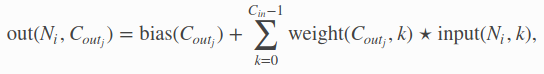# Why do we need out_channels argument in Conv1d()?

``````>>> input = torch.ones(1, 2 , 5)
>>> m = nn.Conv1d(2, 3, 3)
>>> m(input).shape
torch.Size([1, 3, 3])
``````

C_in = 2, C_out = 3
Why do we need that argument? i thought that 1Dconv try to work on every vector(channel) separately and apply 1D filter on it producing the same number of channels. Does this function use a separate 2D filter for that purpose(changing the channel size)?No. I think what you thought is wrong.
What Conv1D does is the same as Conv2D.
So Conv2D takes input of [batch_size x depth x width x height] and output [batch_size x new_depth x (maybe-new)width x (maybe-new)height]. Here c_in = depth and c_out = new_depth. The parameters have the size of depth x new_depth x kernel_size, so you have to specify all these numbers to create the layer.
Conv1D is the same, except that the height dimension size is now always 1, you can think of it that way.

Each filter work on all channels (depth dimension), but only locally in the spatial dimension (width & height) according to the kernel size, so having size [depth x kernel_size]. There is new_depth of those filters. So the parameters are [new_depth x depth x kernel_size] (or more if there’s bias)

1 Like